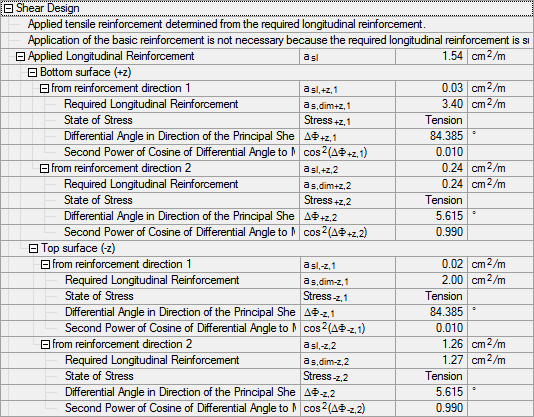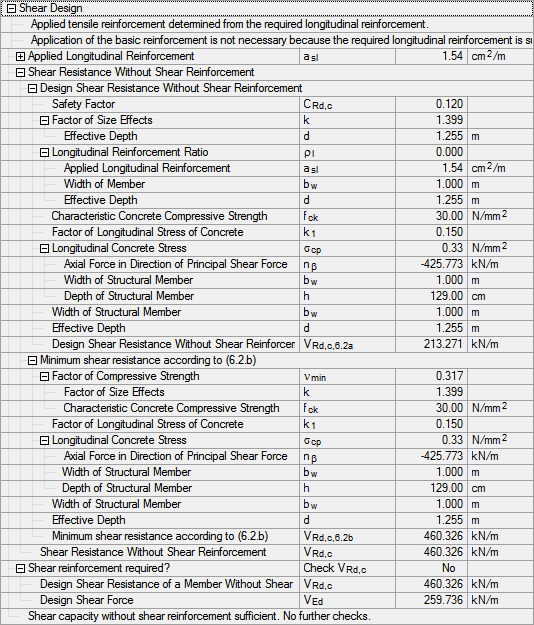# RF-CONCRETE Surfaces – Online Manual Version 5

Online manuals, introductory examples, tutorials, and other documentation.

# 2.5.6 Shear Design

### Shear Design

In the shear design, the applied tensile reinforcement is determined first.Figure 2.74 Applied tensile reinforcement

From all reinforcement layers and directions, a total of 1.54 cm2/m of tension reinforcement can be applied. With it, the shear force VRd,c that can be resisted without shear reinforcement is determined.Figure 2.75 Design shear resistance without shear reinforcement

With the applied tensile reinforcement, the longitudinal reinforcement ratio ρl is determined:

In a 3D model type (in contrast to a plate), an additional axial force can occur. It must be considered via the corresponding concrete longitudinal stress.

The factor k for considering the plate thickness is calculated as follows:

The following factors are also included in the design:

 Factor of concrete longitudinal stress k1 = 0.15 Concrete compressive strength for C30/37 fck = 30.0 N/mm2 Safety factor

Thus, the design shear resistance VRd,c without shear reinforcement can be determined according to Equation (6.2a):

According to Equation (6.2b), the minimum value of the design shear resistance VRd,c without shear reinforcement is determined from the minimum reinforcement ratio νmin:

Because the plate's design shear resistance VRd,c = 459.96 kN/m is greater than the applied shear force VEd = 259.726 kN/m, no shear reinforcement is required in the example.

Should the plate's shear resistance be insufficient, the program first checks if the maximum shear resistance of the concrete compression strut VRd,max is sufficient. VRd,max is determined with the minimum inclination of the compression strut θ. When the design shear resistance of the concrete compression strut is greater than the applied shear force VEd, the statically required shear reinforcement req asw can be determined. Then the design for the shear reinforcement VRd,sy is carried out.